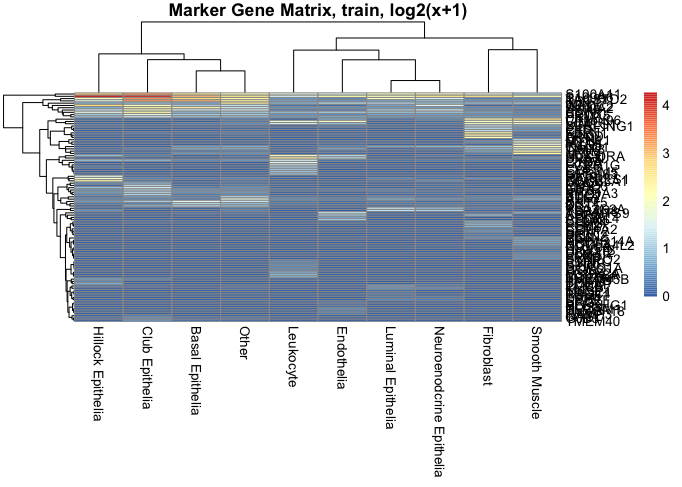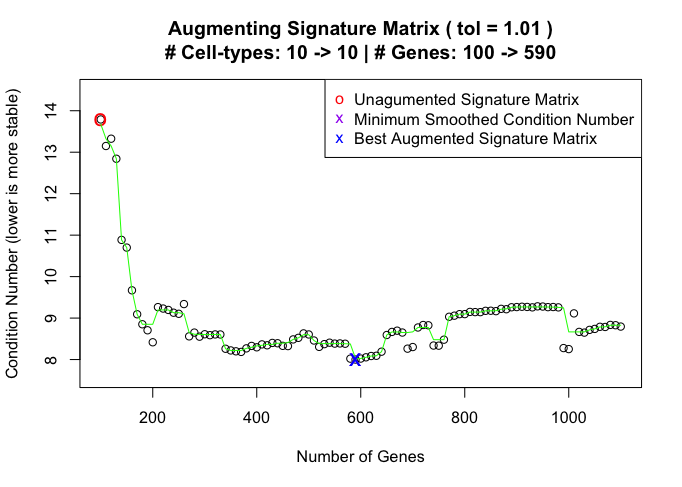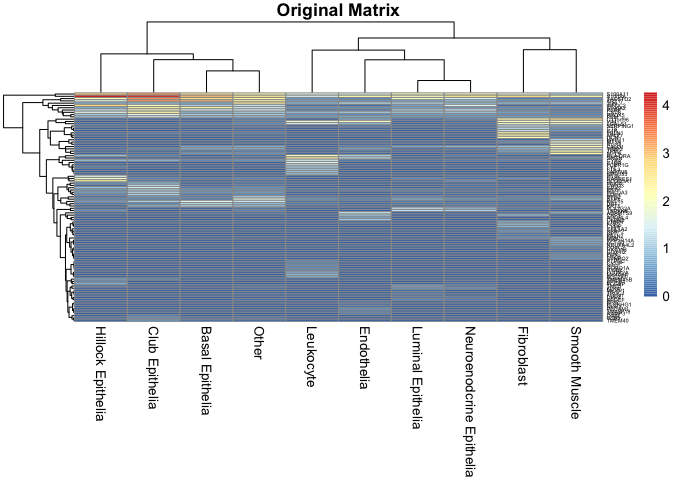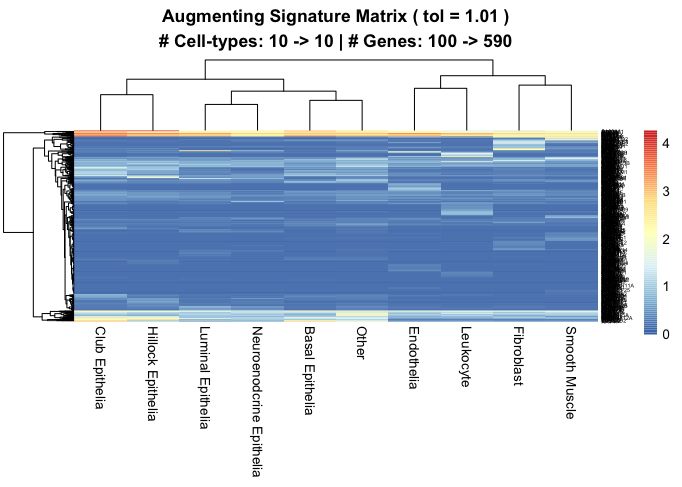install.packages(‘devtools’)

library(devtools)

``````library(ADAPTS)
library(pheatmap)
library(parallel)
library(ranger)
doParallel::registerDoParallel(cores = parallel::detectCores())
set.seed(42)``````

### Step 1: Build signature matrices from the scHenry data

This prostate data set comes from Henry, Gervaise H., et al. “A cellular anatomy of the normal adult human prostate and prostatic urethra.” Cell reports 25.12 (2018): 3530-3542.

``scHenry<-log(ADAPTSdata4::scHenry+1)``

#### Step 1a: Randomly split the dataset into training and test set

Around half of the cell types fall into the training set and the other half fall into the test set. scSample function helps collapse cell types with similar cell type IDs into n (groupsize) groups.

``trainTestSet<-ADAPTS::splitSCdata(scHenry,numSets = 2,randomize = TRUE)``
``## Basal Epithelia : 18439; 9220, 9219``
``## Other : 7163; 3582, 3581``
``## Luminal Epithelia : 2238; 1119, 1119``
``## Fibroblast : 1168; 584, 584``
``## Hillock Epithelia : 1312; 656, 656``
``## Smooth Muscle : 945; 473, 472``
``## Endothelia : 1586; 793, 793``
``## Leukocyte : 459; 230, 229``
``## Club Epithelia : 2530; 1265, 1265``
``## Neuroenodcrine Epithelia : 25; 13, 12``
``````trainSet<-as.matrix(trainTestSet[])
testSet<-as.matrix(trainTestSet[])

trainSet.30sam <- ADAPTS::scSample(RNAcounts = trainSet, groupSize = 30, randomize = TRUE)
trainSet.3sam <- ADAPTS::scSample(RNAcounts = trainSet, groupSize = 3, randomize = TRUE)``````

#### Step 1b: Build a deconvolution seed matrix using ranger forest and estimate the accuracy on pseudo bulk test set

ADAPTS provides the option of building a new seed matrix de novo based on the sample given, in addition to augmenting existing signature matrices, such as LM22. This is particularly helpful for single cell data sets, where the cell types present have come from their native tissue.

``seedMat<-ADAPTS::buildSeed(trainSet=trainSet, trainSet.3sam = trainSet.3sam, trainSet.30sam = trainSet.30sam, genesInSeed = 100, groupSize = 30, randomize = TRUE, num.trees = 1000, plotIt = TRUE)````````pseudobulk.test <- data.frame(test=rowSums(testSet))
pseudobulk.test.counts<-table(colnames(testSet))
actFrac.test <- 100 * pseudobulk.test.counts / sum(pseudobulk.test.counts)

colnames(estimates.test)<-'seed'
estimates.test\$actFrac<-round(actFrac.test[rownames(estimates.test)],2)

#### Step 1c: Build a deconvolution matrix using all the genes and estimate the accuracy on pseudo bulk test set

This step tests if building signature matrix is really necessary by comparing the performance of signature matrices and all-gene matrix.

``````allGeneSig <- apply(trainSet.3sam, 1, function(x){tapply(x, colnames(trainSet.3sam), mean, na.rm=TRUE)})

colnames(estimates.allGene)<-'all'
estimates.test<-cbind(estimates.allGene,estimates.test)

#### Step 1d: Augment the seed matrix and estimate the accuray on pseudo bulk test set

ADAPTS takes in the seed matrix, adds one additional gene from the full data at a time and records their condition number. The new augmented signature matrix is chosen based on the lowest condition number.

``````gList <- ADAPTS::gListFromRF(trainSet=trainSet.30sam)
augTrain <- ADAPTS::AugmentSigMatrix(origMatrix = seedMat, fullData = trainSet.3sam, gList = gList, nGenes = 1:100, newData = trainSet.3sam, plotToPDF = FALSE, pdfDir = '.')````````````estimates.augment <- as.data.frame(ADAPTS::estCellPercent.DCQ(augTrain, pseudobulk.test))
colnames(estimates.augment) <- 'aug'
estimates.test <- cbind(estimates.augment, estimates.test)

``augTrain.shrink <- ADAPTS::shrinkSigMatrix(augTrain, numChunks=NULL, verbose=FALSE, plotIt=TRUE, sigGenesList=NULL, singleCore=FALSE, fastStop=FALSE)``
``````## Registered S3 method overwritten by 'quantmod':